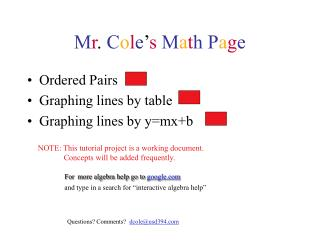Download PresentationM r . C o l e ’ s M a t h P a g eM r . C o l e ’ s M a t h P a g e - PowerPoint PPT Presentation

Download PresentationM r . C o l e ’ s M a t h P a g e
An Image/Link below is provided (as is) to download presentation

Download Policy: Content on the Website is provided to you AS IS for your information and personal use and may not be sold / licensed / shared on other websites without getting consent from its author. While downloading, if for some reason you are not able to download a presentation, the publisher may have deleted the file from their server.

- - - - - - - - - - - - - - - - - - - - - - - - - - - E N D - - - - - - - - - - - - - - - - - - - - - - - - - - -
Presentation Transcript

1. Mr. Cole’sMathPage • Ordered Pairs • Graphing lines by table • Graphing lines by y=mx+b NOTE: This tutorial project is a working document. Concepts will be added frequently. Formore algebra help go to google.com and type in a search for “interactive algebra help” Questions? Comments? dcole@usd394.com

2. This concept will be well explained when you click on the link to “Math Dork.Com” Enter Home

3. In order to graph by table we need several things: • First you need an equation, lets try y=2x-4 • Next we need to have a table of values. • Click for the table of values. Home

4. In order to graph by table we need several things: • First you need an equation, lets try y=2x-4 • Next we need to have a table of values. x y= 2x - 4 0 2(0) - 4 = -4 1 2(1) - 4 = -2 2 2(2) - 4 = 0 3 2(3) - 4 = 2 Click to see graph Home

5. Plotting the points one at a time. x y= 2x - 4 0 2(0) - 4 = -4 (3,2) 1 2(1) - 4 = -2 (2,0) 2 2(2) - 4 = 0 (1,-2) 3 2(3) - 4 = 2 (0,-4) Next we will plot the ordered pairs on the cartesian coordinate plane. See that again Another example More on this from the internet

6. Plotting the points one at a time. x y= 2x - 4 (3,2) 0 2(0) - 4 = -4 (2,0) 1 2(1) - 4 = -2 2 2(2) - 4 = 0 (1,-2) 3 2(3) - 4 = 2 (0,-4) Next we will plot the ordered pairs on the cartesian coordinate plane. See that again Another example More on this from the internet

7. Plotting the points one at a time. x y= 2x - 4 (3,2) 0 2(0) - 4 = -4 (2,0) 1 2(1) - 4 = -2 2 2(2) - 4 = 0 (1,-2) 3 2(3) - 4 = 2 (0,-4) Next we will plot the ordered pairs on the cartesian coordinate plane. Another example More on this from the internet

8. This time let’s try the equation y=-3x+5 • Again we need a table of values. (0,5) x y= -3x+5 (1,2) 0 -3(0)+5 = 5 1 -3(1)+5 = 2 (2,-1) 2 -3(2)+5 = -1 3 -3(3)+5 = -4 (3,-4) Next we will plot the ordered pairs on the cartesian coordinate plane See graph

9. Plotting the points in red will generate The line (0,5) x y= -3x+5 (1,2) 0 -3(0)+5 = 5 1 -3(1)+5 = 2 (2,-1) 2 -3(2)+5 = -1 3 -3(3)+5 = -4 (3,-4) See that again Home For more information from the internet

10. Plotting the points in red will generate The line (0,5) x y= -3x+5 (1,2) 0 -3(0)+5 = 5 1 -3(1)+5 = 2 (2,-1) 2 -3(2)+5 = -1 3 -3(3)+5 = -4 (3,-4) For more on this topic From the internet. Home

11. Plotting the points in red will generate The line (0,5) x y= -3x+5 (1,2) 0 -3(0)+5 = 5 1 -3(1)+5 = 2 (2,-1) 2 -3(2)+5 = -1 3 -3(3)+5 = -4 (3,-4) For more on this topic From the internet. Home

12. Graphing by y=mx+b m is the slope of the line b is the y-intercept Review these Concepts • slope = rise • run • Y-intercept means where the line CROSSES the y-axis • When graphing the line it is important to remember • to plot the y-intercept FIRST, then the slope!

13. For example, we want to graph y=(4/3)x-2 The slope is 4/3 (up 4 over 3) Over 3 Up 4 The y-intercept is -2 (crosses the y-axis at -2) Graph the And remember, ! y-intercept First Another example For Interactive practice from Mr. Bunge Home

14. Remember the equation was y=(4/3)x-2 The slope is 4/3 (up 4 over 3) Over 3 Up 4 The y-intercept is -2 (crosses the y-axis at -2) For another example For Interactive practice from Mr. Bunge

15. This time lets try to graph y=-3x+2 The slope is -3 (down 3 over 1) Down 3 The y-intercept is 2 (crosses the y-axis at 2) Over 1 Again, graph the y-intercept first ! For Interactive practice from Mr. Bunge Home

16. Remember the equation was y=(4/3)x-2 The slope is 4/3 (up 4 over 3) Over 3 The y-intercept is -2 (crosses the y-axis at -2) Up 4 For another example

17. Click on one of the following to review this concept from the internet. Summary of lines PurpleMath.Com Home Questions? Comments? dcole@usd394.com